# Intermolecular Forces and Liquids and Solids Chapter 11

• Slides: 58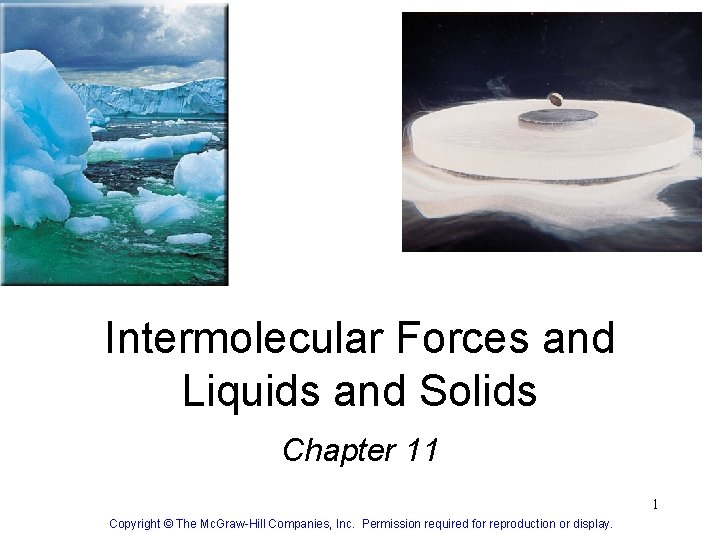Intermolecular Forces and Liquids and Solids Chapter 11 1 Copyright © The Mc. Graw-Hill Companies, Inc. Permission required for reproduction or display.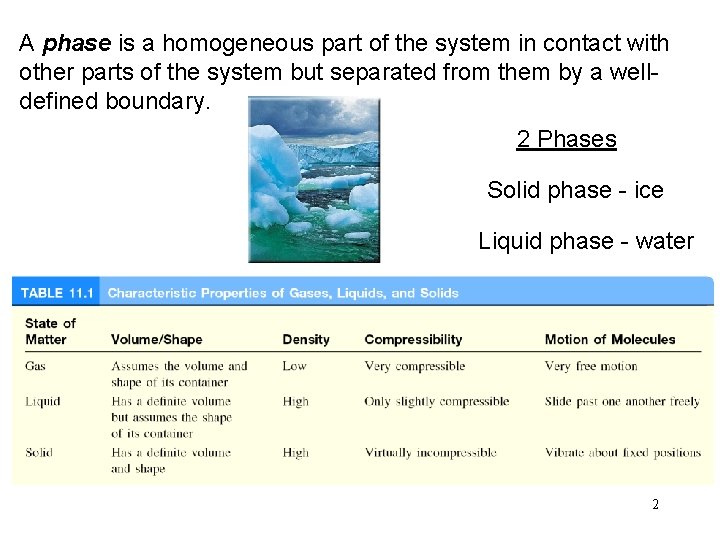A phase is a homogeneous part of the system in contact with other parts of the system but separated from them by a welldefined boundary. 2 Phases Solid phase - ice Liquid phase - water 2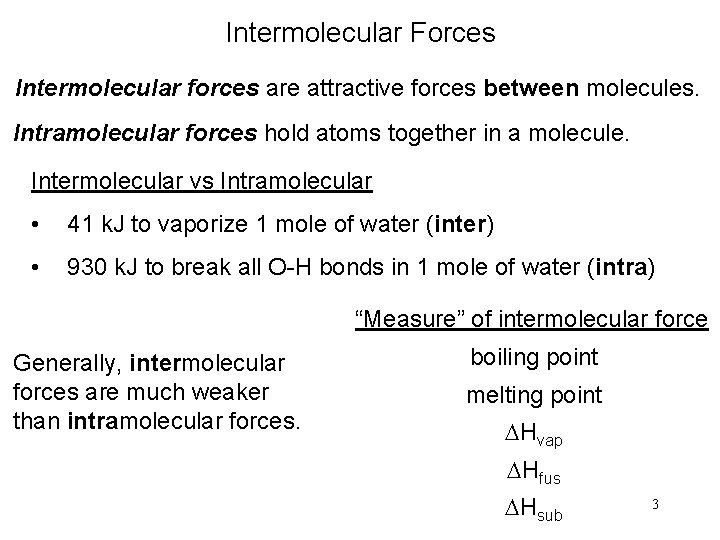Intermolecular Forces Intermolecular forces are attractive forces between molecules. Intramolecular forces hold atoms together in a molecule. Intermolecular vs Intramolecular • 41 k. J to vaporize 1 mole of water (inter) • 930 k. J to break all O-H bonds in 1 mole of water (intra) “Measure” of intermolecular force Generally, intermolecular forces are much weaker than intramolecular forces. boiling point melting point DHvap DHfus DHsub 3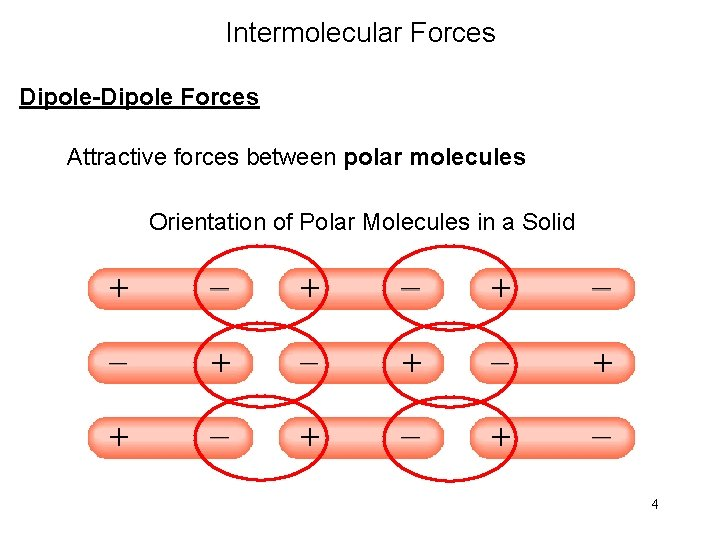Intermolecular Forces Dipole-Dipole Forces Attractive forces between polar molecules Orientation of Polar Molecules in a Solid 4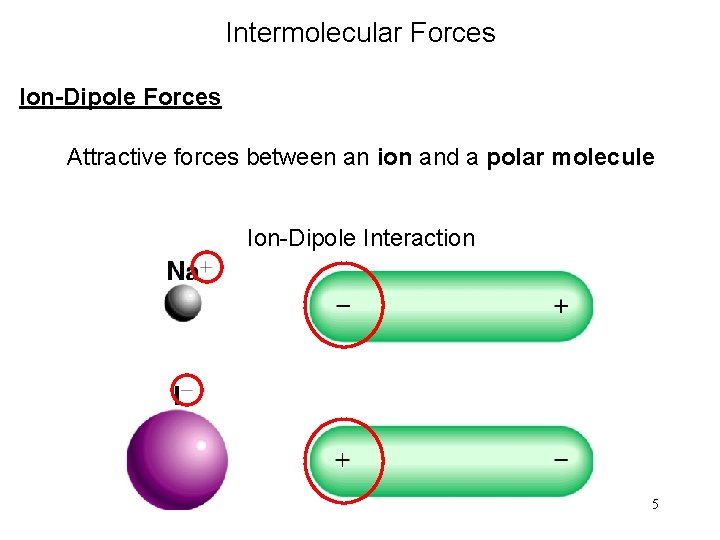Intermolecular Forces Ion-Dipole Forces Attractive forces between an ion and a polar molecule Ion-Dipole Interaction 5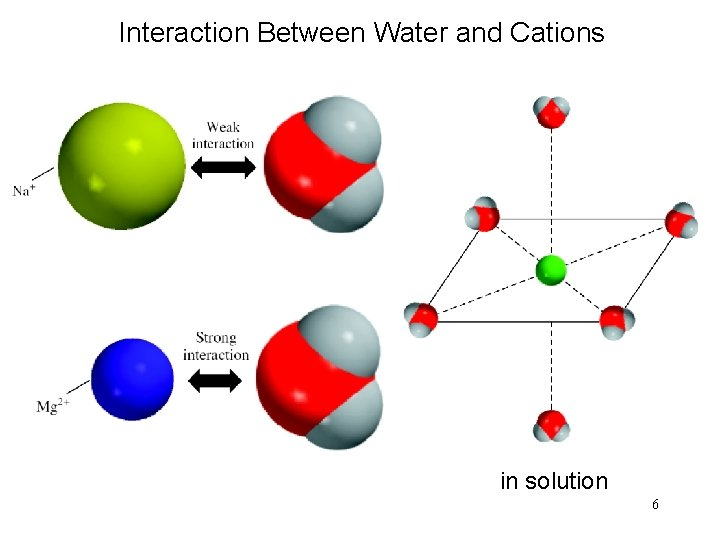Interaction Between Water and Cations in solution 6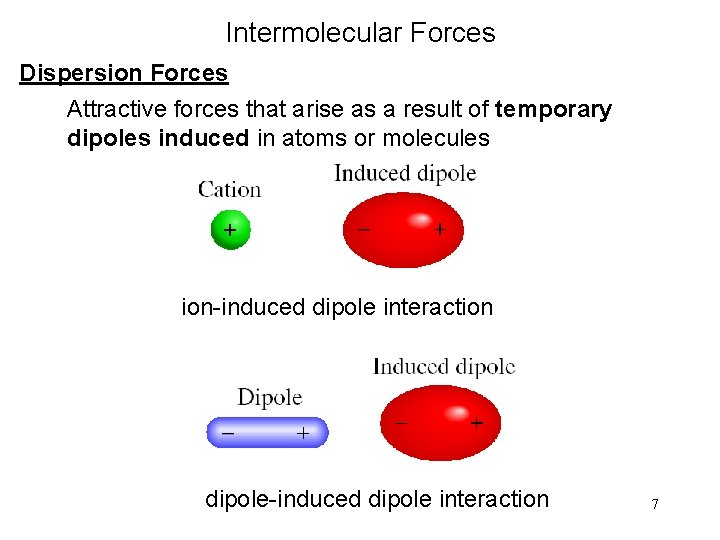Intermolecular Forces Dispersion Forces Attractive forces that arise as a result of temporary dipoles induced in atoms or molecules ion-induced dipole interaction dipole-induced dipole interaction 7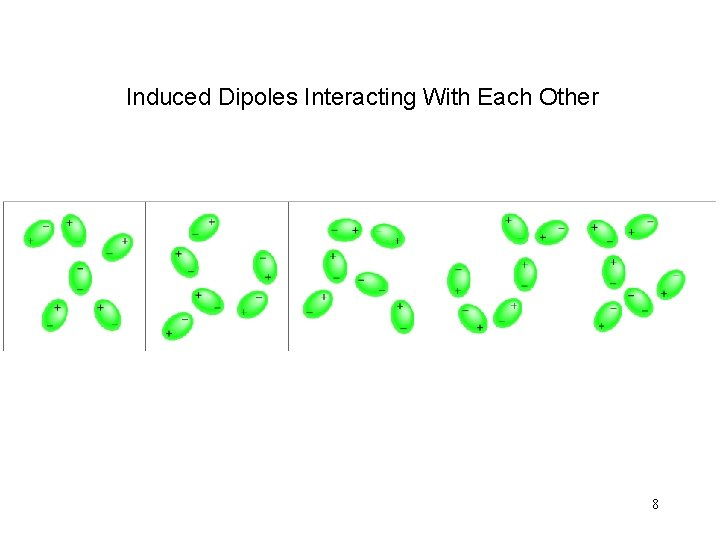Induced Dipoles Interacting With Each Other 8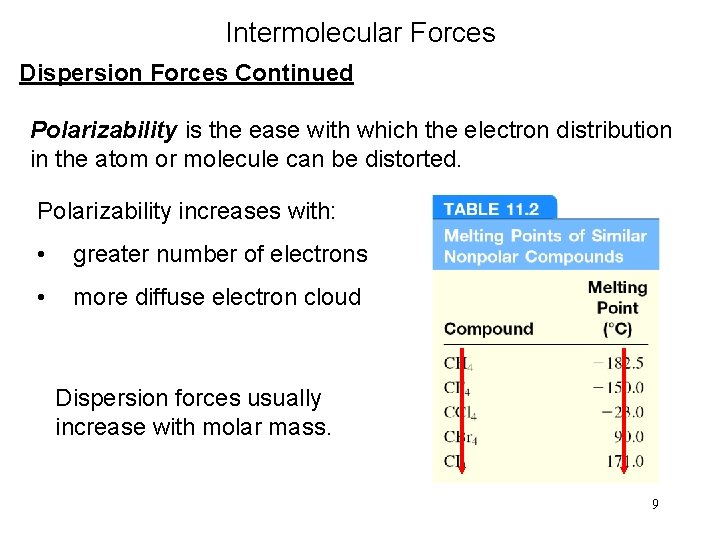Intermolecular Forces Dispersion Forces Continued Polarizability is the ease with which the electron distribution in the atom or molecule can be distorted. Polarizability increases with: • greater number of electrons • more diffuse electron cloud Dispersion forces usually increase with molar mass. 9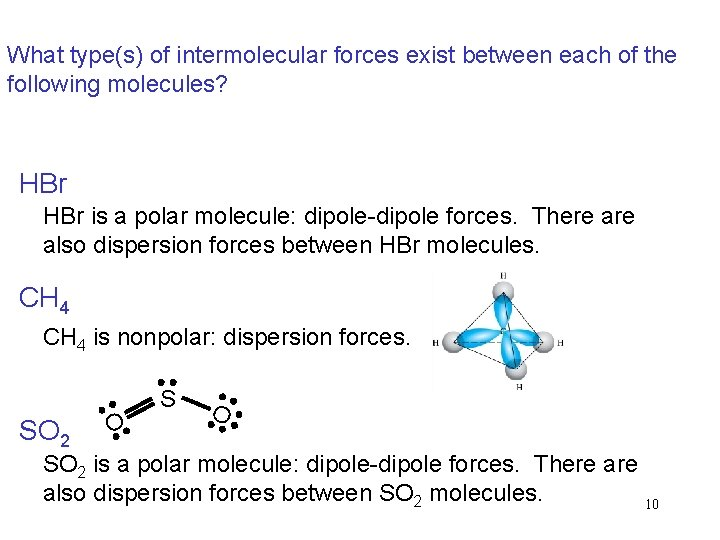What type(s) of intermolecular forces exist between each of the following molecules? HBr is a polar molecule: dipole-dipole forces. There also dispersion forces between HBr molecules. CH 4 is nonpolar: dispersion forces. SO 2 O SO 2 is a polar molecule: dipole-dipole forces. There also dispersion forces between SO 2 molecules. 10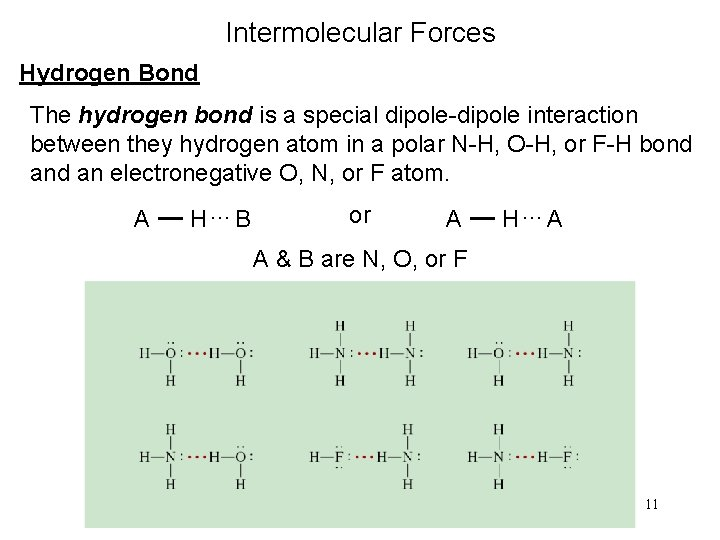Intermolecular Forces Hydrogen Bond The hydrogen bond is a special dipole-dipole interaction between they hydrogen atom in a polar N-H, O-H, or F-H bond an electronegative O, N, or F atom. A H…B or A H…A A & B are N, O, or F 11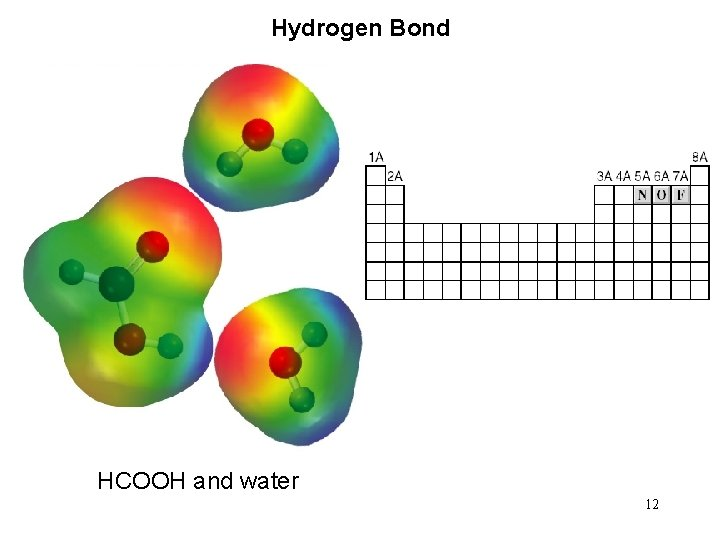Hydrogen Bond HCOOH and water 12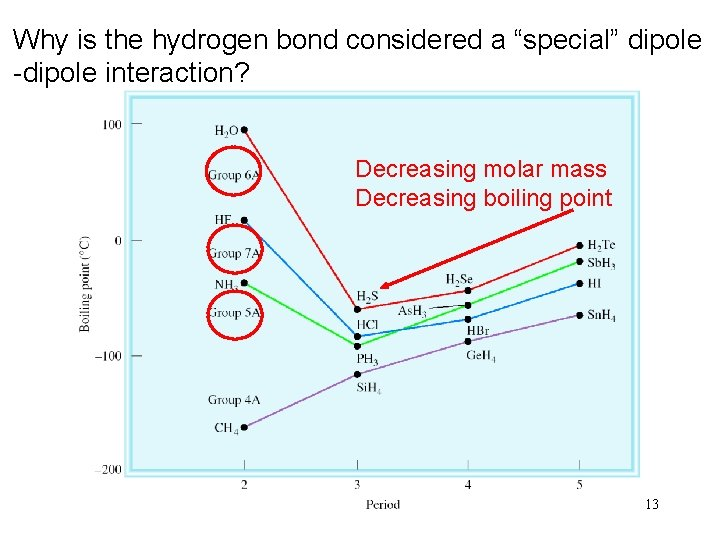Why is the hydrogen bond considered a “special” dipole -dipole interaction? Decreasing molar mass Decreasing boiling point 13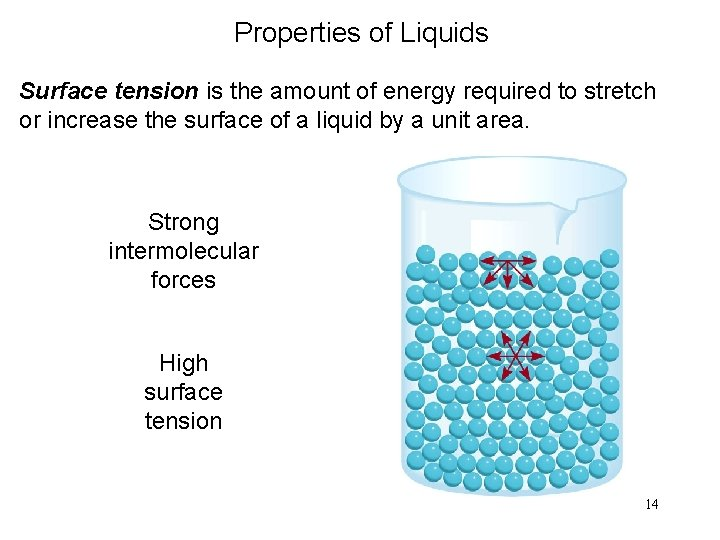Properties of Liquids Surface tension is the amount of energy required to stretch or increase the surface of a liquid by a unit area. Strong intermolecular forces High surface tension 14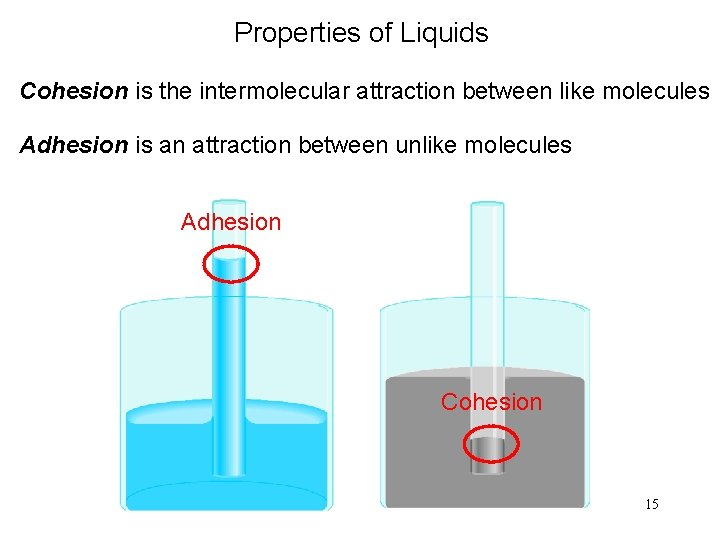Properties of Liquids Cohesion is the intermolecular attraction between like molecules Adhesion is an attraction between unlike molecules Adhesion Cohesion 15Properties of Liquids Viscosity is a measure of a fluid’s resistance to flow. Strong intermolecular forces High viscosity 163 -D Structure of Water is a Unique Substance Maximum Density 4 0 C Density of Water Ice is less dense than water 17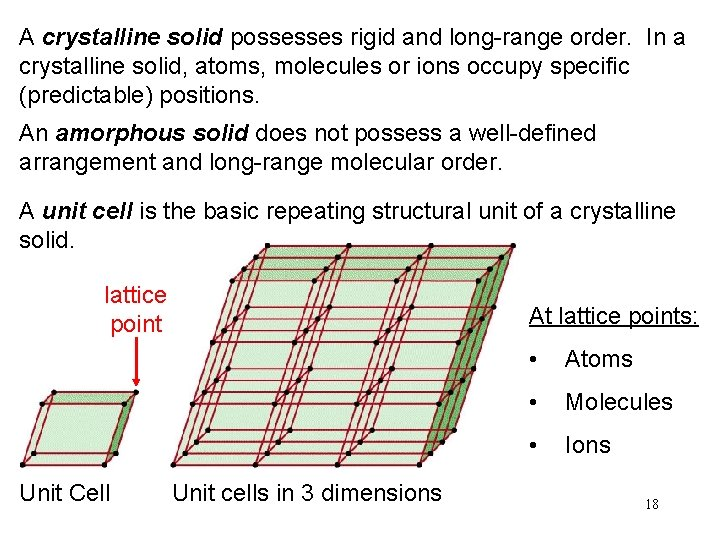A crystalline solid possesses rigid and long-range order. In a crystalline solid, atoms, molecules or ions occupy specific (predictable) positions. An amorphous solid does not possess a well-defined arrangement and long-range molecular order. A unit cell is the basic repeating structural unit of a crystalline solid. lattice point Unit Cell At lattice points: Unit cells in 3 dimensions • Atoms • Molecules • Ions 18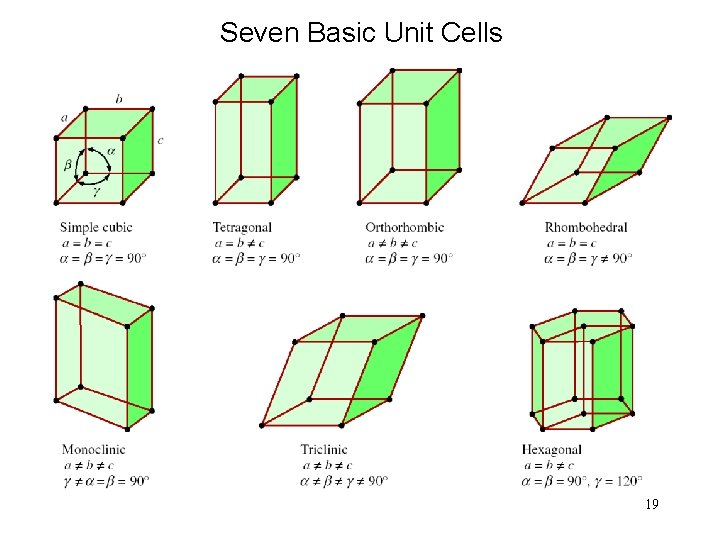Seven Basic Unit Cells 19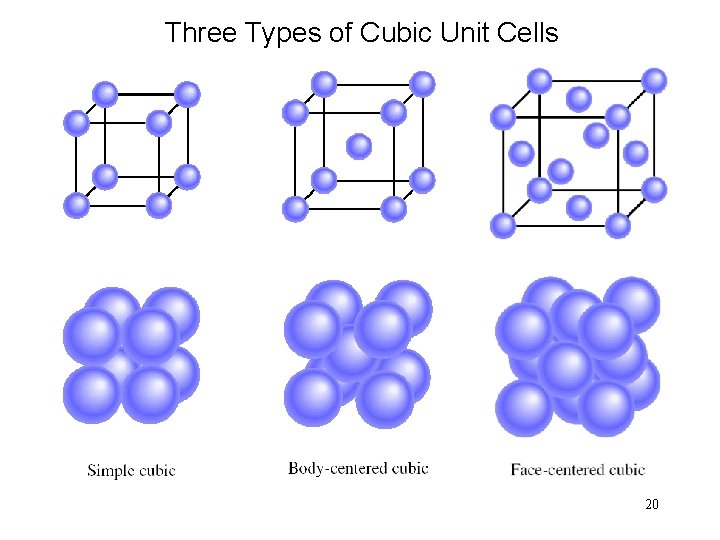Three Types of Cubic Unit Cells 20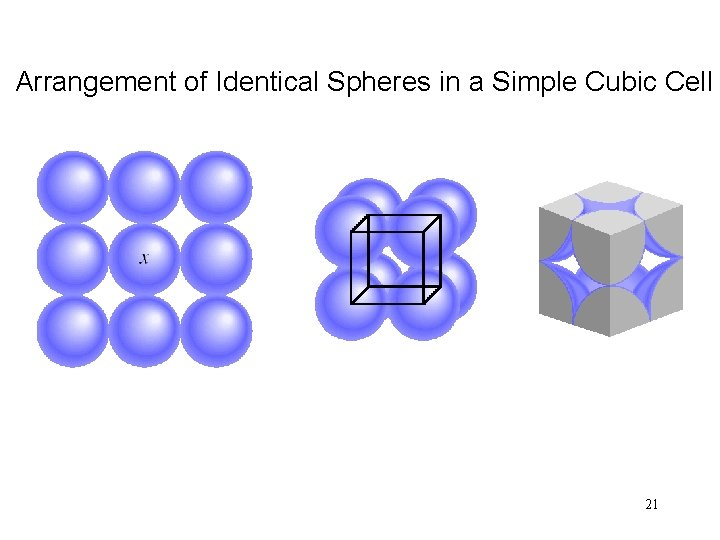Arrangement of Identical Spheres in a Simple Cubic Cell 21Arrangement of Identical Spheres in a Body-Centered Cubic Cell 22A Corner Atom, a Edge-Centered Atom and a Face-Centered Atom Shared by 8 unit cells Shared by 4 unit cells Shared by 2 unit cells 23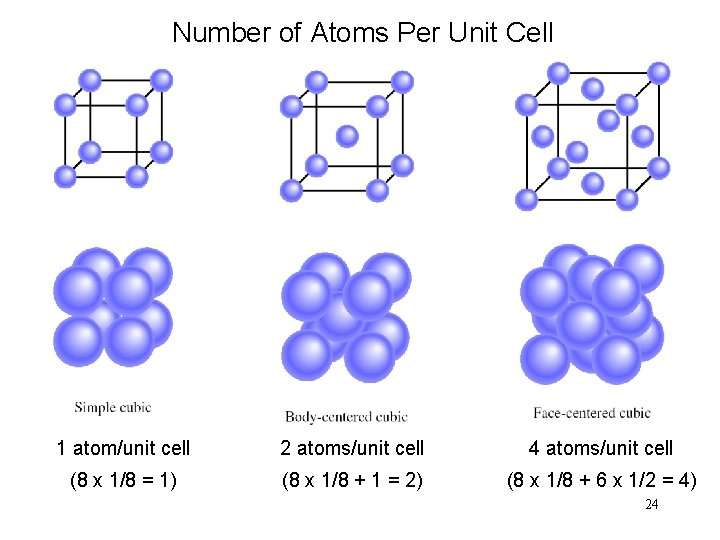Number of Atoms Per Unit Cell 1 atom/unit cell 2 atoms/unit cell 4 atoms/unit cell (8 x 1/8 = 1) (8 x 1/8 + 1 = 2) (8 x 1/8 + 6 x 1/2 = 4) 24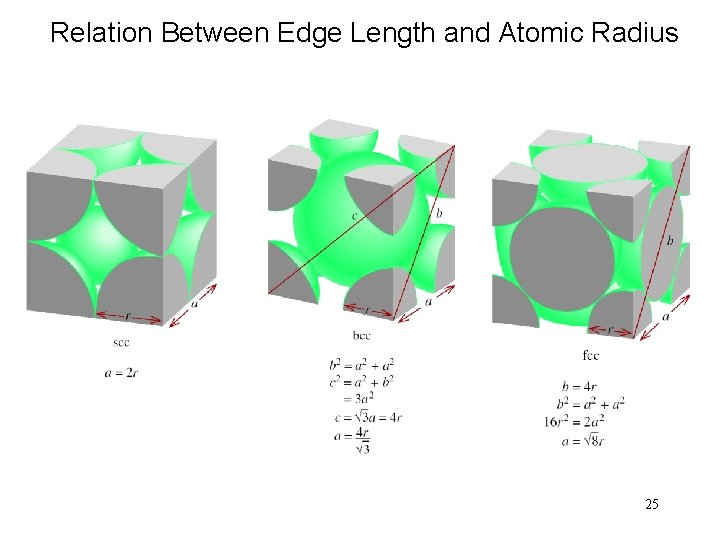Relation Between Edge Length and Atomic Radius 25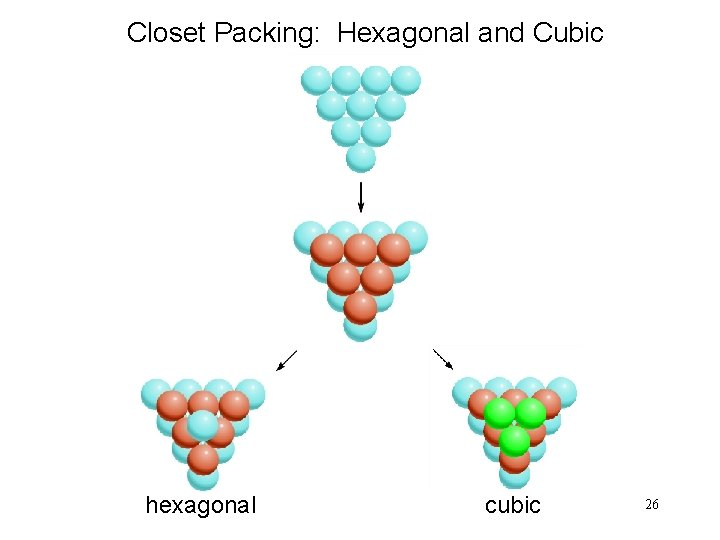Closet Packing: Hexagonal and Cubic hexagonal cubic 26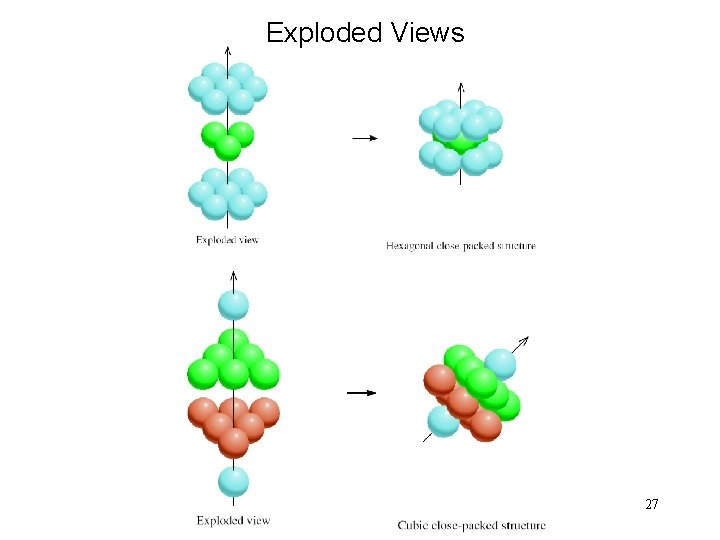Exploded Views 27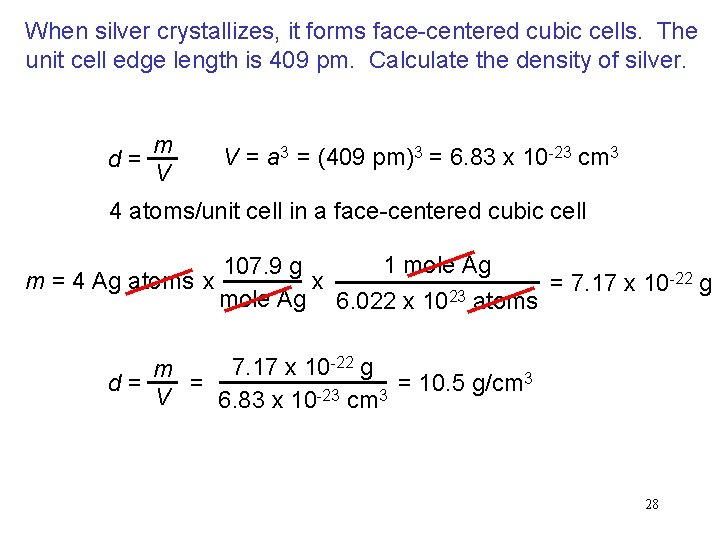When silver crystallizes, it forms face-centered cubic cells. The unit cell edge length is 409 pm. Calculate the density of silver. d = m V V = a 3 = (409 pm)3 = 6. 83 x 10 -23 cm 3 4 atoms/unit cell in a face-centered cubic cell 1 mole Ag 107. 9 g -22 g x m = 4 Ag atoms x = 7. 17 x 10 mole Ag 6. 022 x 1023 atoms 7. 17 x 10 -22 g m 3 = = 10. 5 g/cm d = V 6. 83 x 10 -23 cm 3 28An Arrangement for Obtaining the X-ray Diffraction Pattern of a Crystal. 29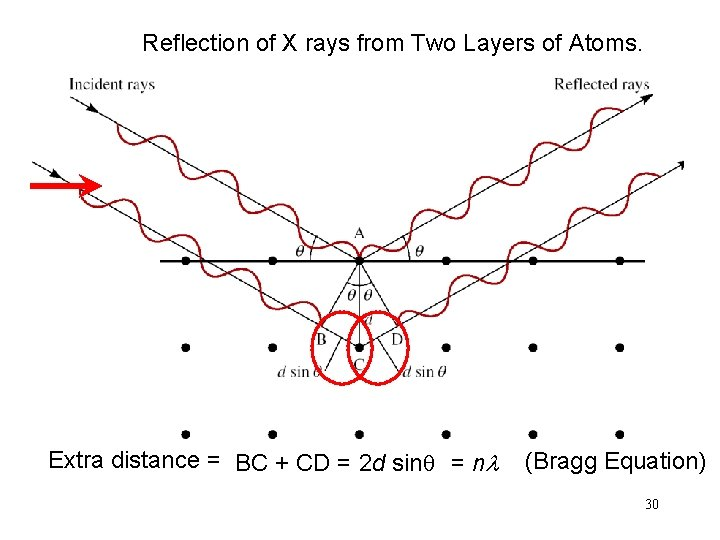Reflection of X rays from Two Layers of Atoms. Extra distance = BC + CD = 2 d sinq = nl (Bragg Equation) 30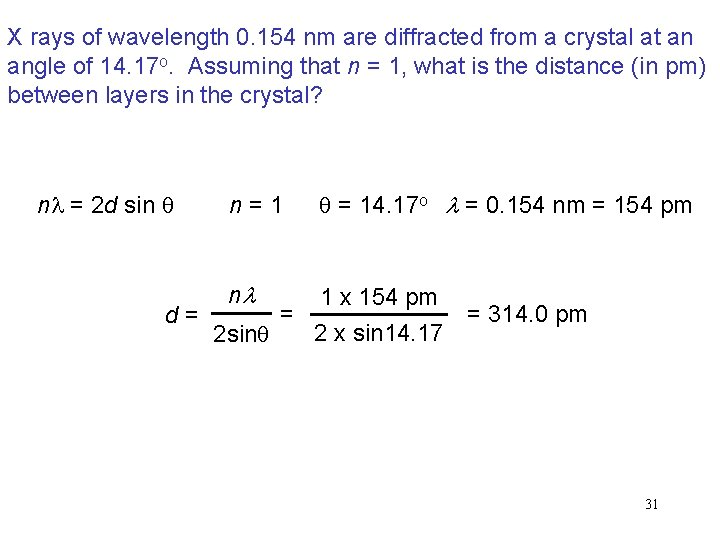X rays of wavelength 0. 154 nm are diffracted from a crystal at an angle of 14. 17 o. Assuming that n = 1, what is the distance (in pm) between layers in the crystal? nl = 2 d sin q n = 1 q = 14. 17 o l = 0. 154 nm = 154 pm nl 1 x 154 pm = = 314. 0 pm d = 2 x sin 14. 17 2 sinq 31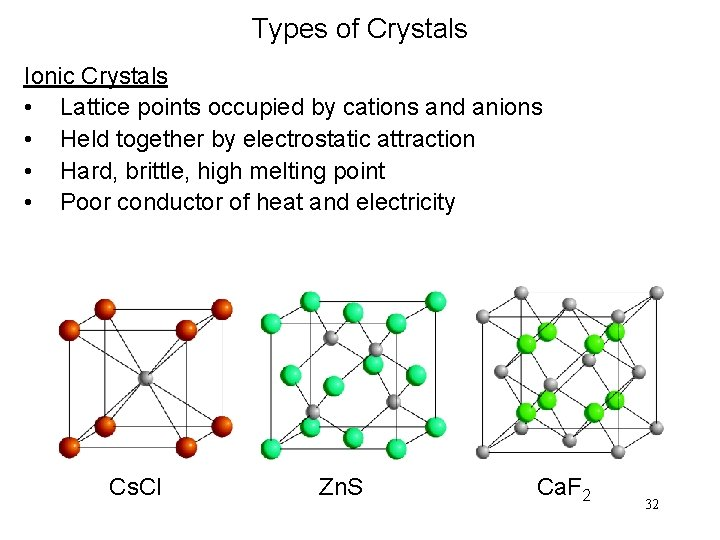Types of Crystals Ionic Crystals • Lattice points occupied by cations and anions • Held together by electrostatic attraction • Hard, brittle, high melting point • Poor conductor of heat and electricity Cs. Cl Zn. S Ca. F 2 32Types of Crystals Covalent Crystals • Lattice points occupied by atoms • Held together by covalent bonds • Hard, high melting point • Poor conductor of heat and electricity carbon atoms diamond graphite 33Types of Crystals Molecular Crystals • Lattice points occupied by molecules • Held together by intermolecular forces • Soft, low melting point • Poor conductor of heat and electricity water benzene 34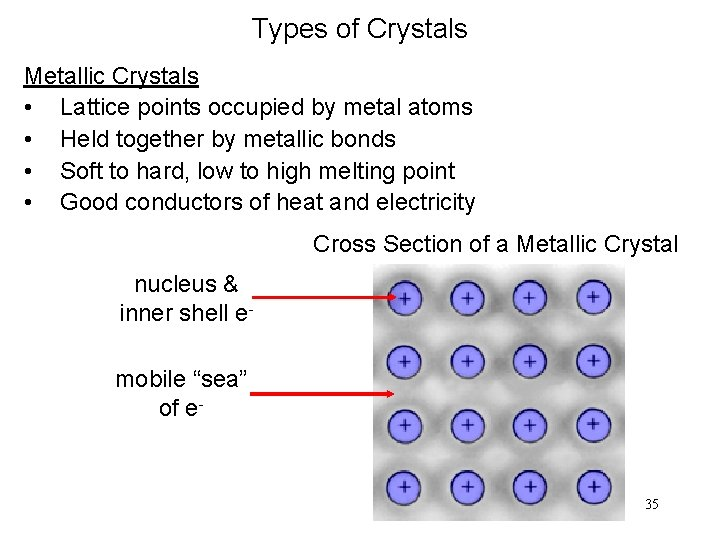Types of Crystals Metallic Crystals • Lattice points occupied by metal atoms • Held together by metallic bonds • Soft to hard, low to high melting point • Good conductors of heat and electricity Cross Section of a Metallic Crystal nucleus & inner shell emobile “sea” of e- 35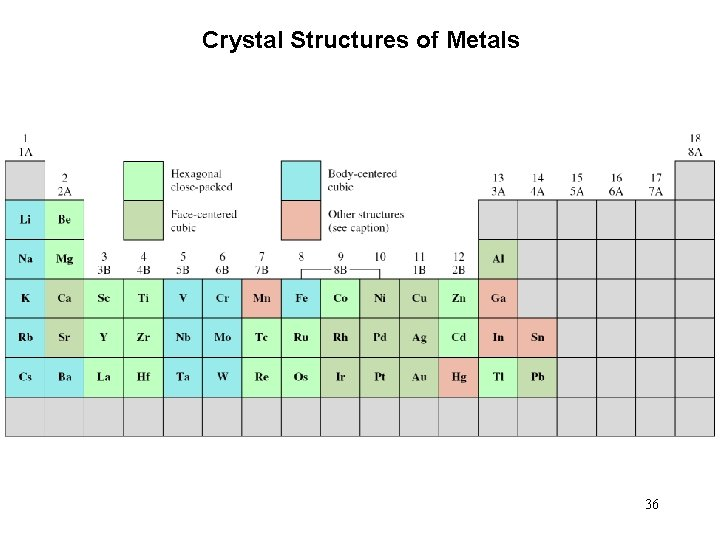Crystal Structures of Metals 36Types of Crystals 37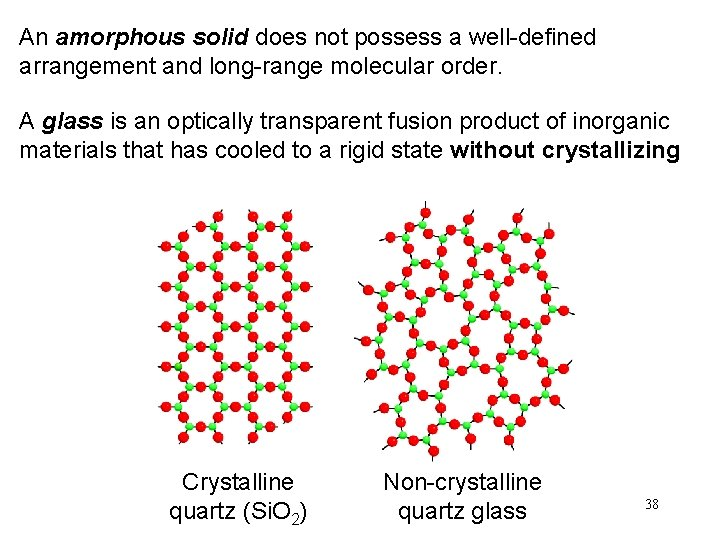An amorphous solid does not possess a well-defined arrangement and long-range molecular order. A glass is an optically transparent fusion product of inorganic materials that has cooled to a rigid state without crystallizing Crystalline quartz (Si. O 2) Non-crystalline quartz glass 38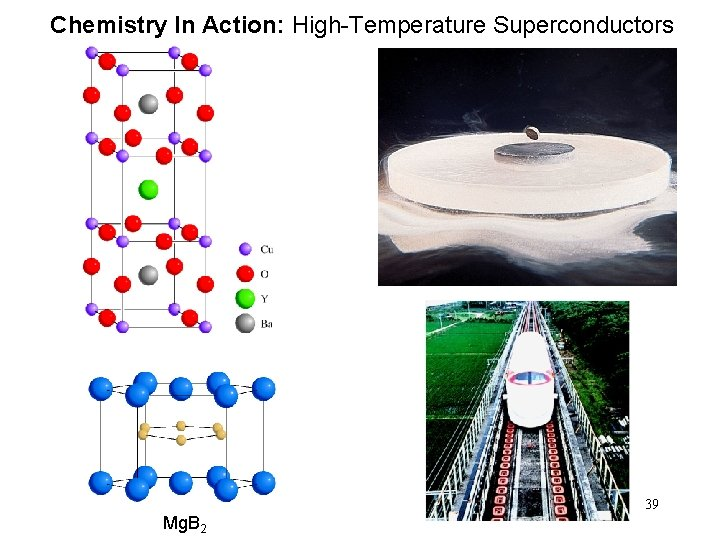Chemistry In Action: High-Temperature Superconductors 39 Mg. B 2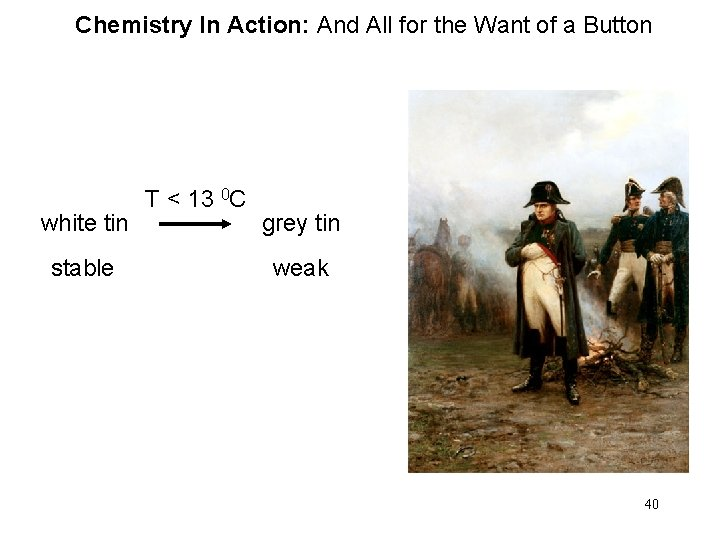Chemistry In Action: And All for the Want of a Button T < 13 0 C white tin grey tin stable weak 40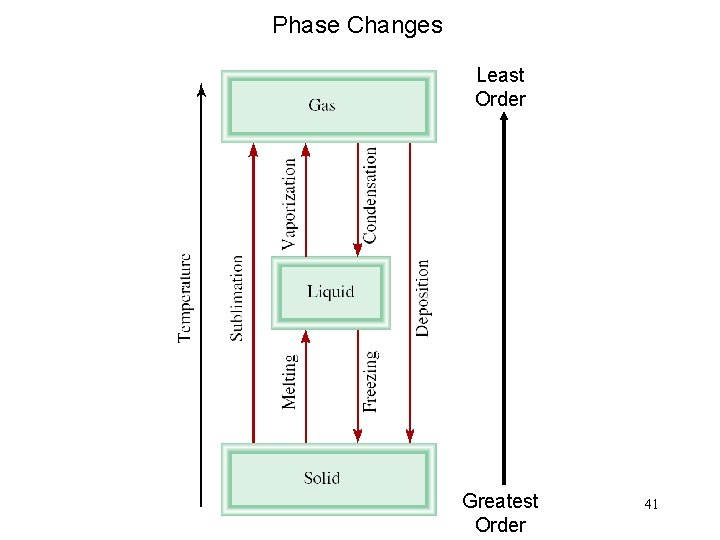Phase Changes Least Order Greatest Order 41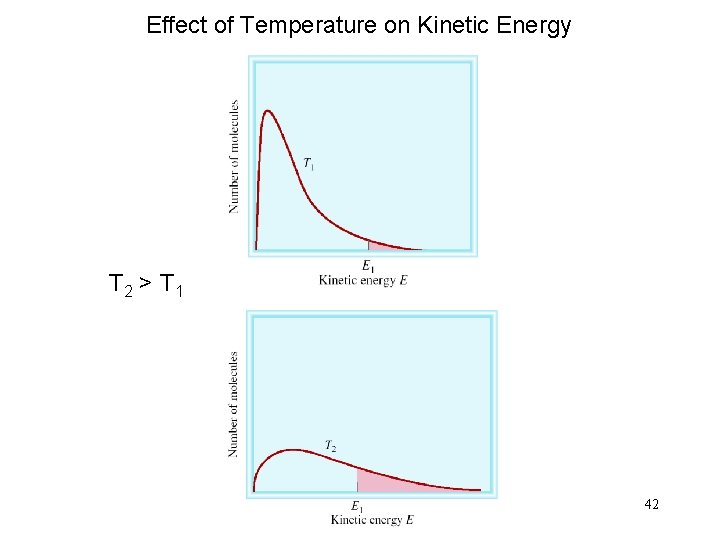Effect of Temperature on Kinetic Energy T 2 > T 1 42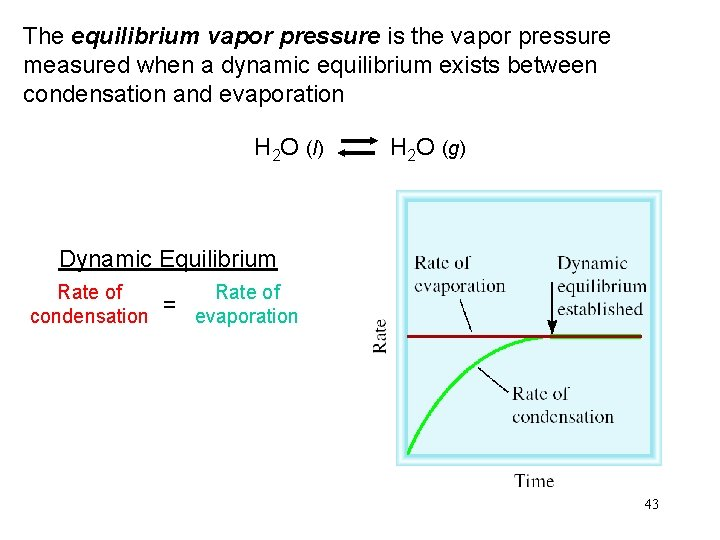The equilibrium vapor pressure is the vapor pressure measured when a dynamic equilibrium exists between condensation and evaporation H 2 O (l) H 2 O (g) Dynamic Equilibrium Rate of condensation = Rate of evaporation 43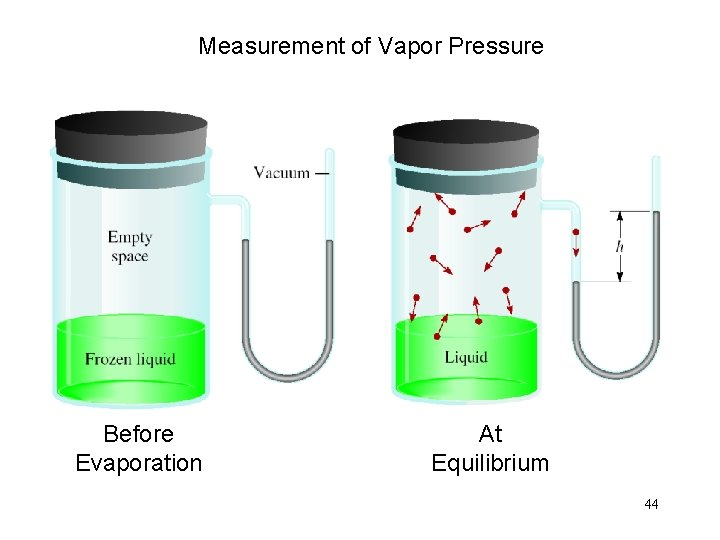Measurement of Vapor Pressure Before Evaporation At Equilibrium 44Molar heat of vaporization (DHvap) is the energy required to vaporize 1 mole of a liquid at its boiling point. Clausius-Clapeyron Equation ln P = - DHvap RT + C P = (equilibrium) vapor pressure T = temperature (K) R = gas constant (8. 314 J/K • mol) Vapor Pressure Versus Temperature 45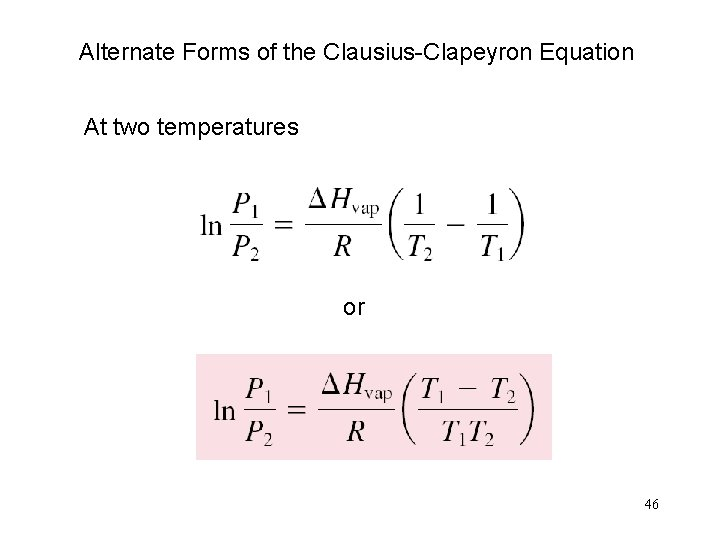Alternate Forms of the Clausius-Clapeyron Equation At two temperatures or 46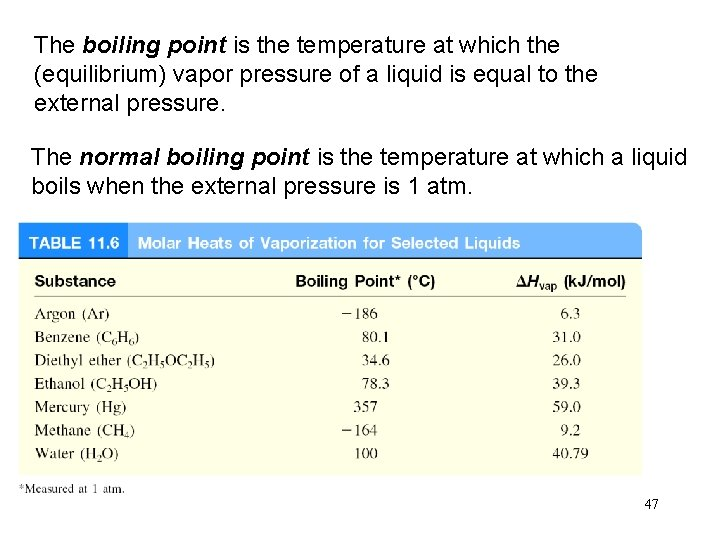The boiling point is the temperature at which the (equilibrium) vapor pressure of a liquid is equal to the external pressure. The normal boiling point is the temperature at which a liquid boils when the external pressure is 1 atm. 47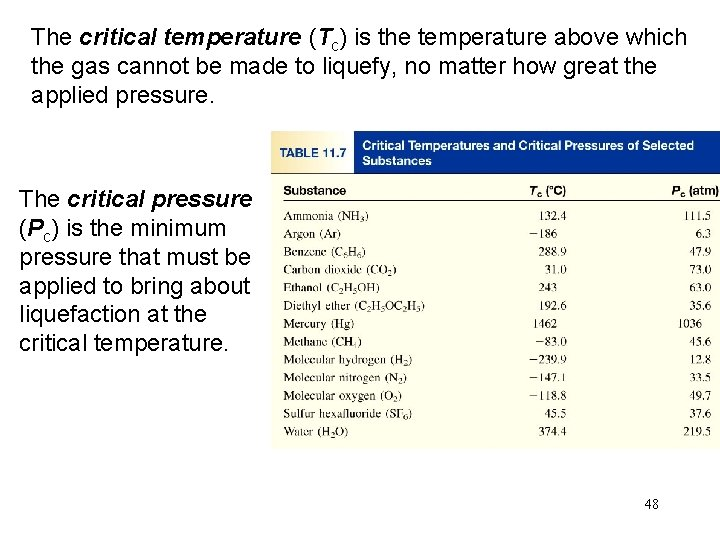The critical temperature (Tc) is the temperature above which the gas cannot be made to liquefy, no matter how great the applied pressure. The critical pressure (Pc) is the minimum pressure that must be applied to bring about liquefaction at the critical temperature. 48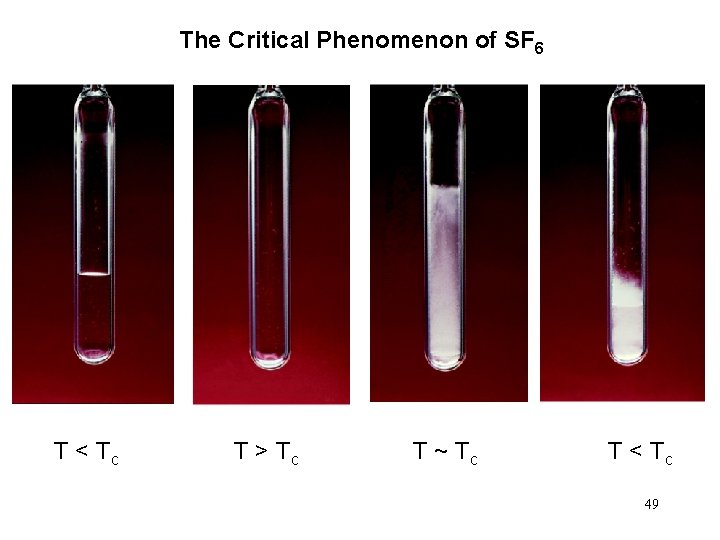The Critical Phenomenon of SF 6 T < Tc T > Tc T ~ Tc T < Tc 49Solid-Liquid Equilibrium H 2 O (s) H 2 O (l) The melting point of a solid or the freezing point of a liquid is the temperature at which the solid and liquid phases coexist in equilibrium 50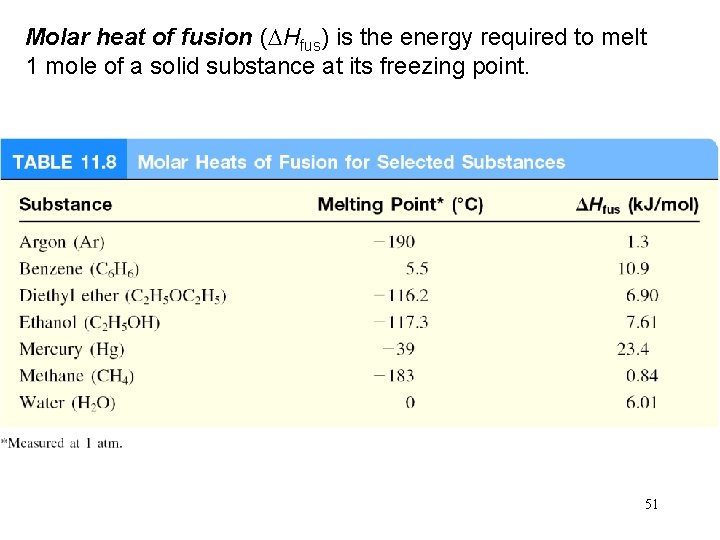Molar heat of fusion (DHfus) is the energy required to melt 1 mole of a solid substance at its freezing point. 51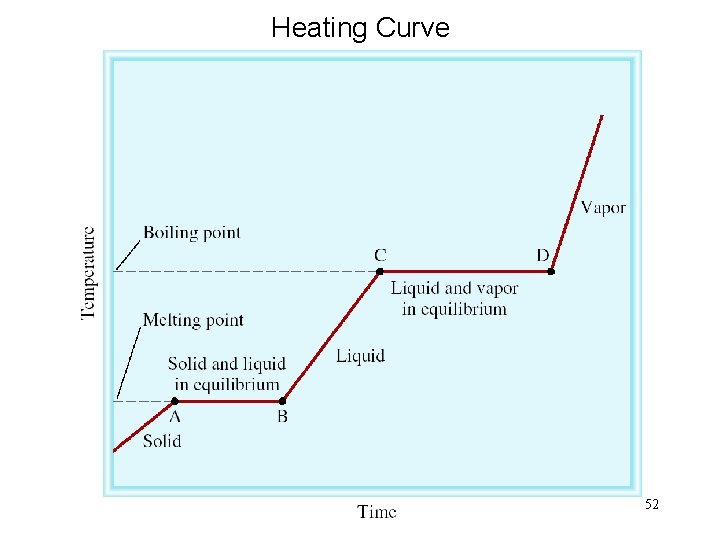Heating Curve 52Solid-Gas Equilibrium H 2 O (s) H 2 O (g) Molar heat of sublimation (DHsub) is the energy required to sublime 1 mole of a solid. DHsub = DHfus + DHvap ( Hess’s Law) 53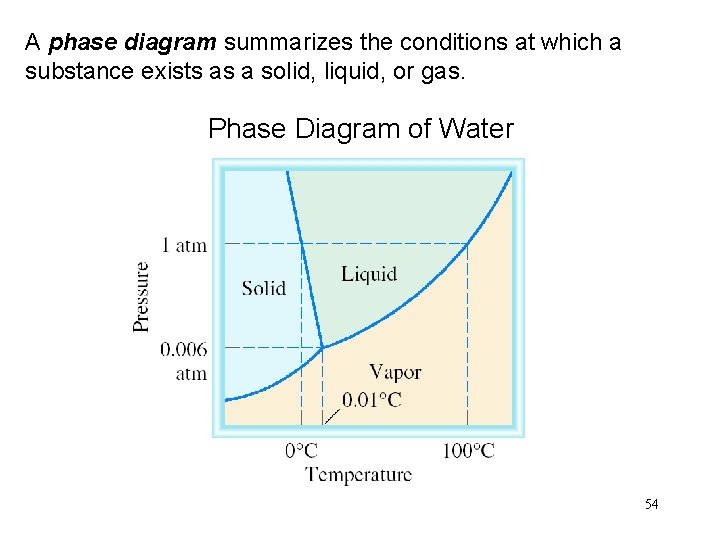A phase diagram summarizes the conditions at which a substance exists as a solid, liquid, or gas. Phase Diagram of Water 54Phase Diagram of Carbon Dioxide At 1 atm CO 2 (s) CO 2 (g) 55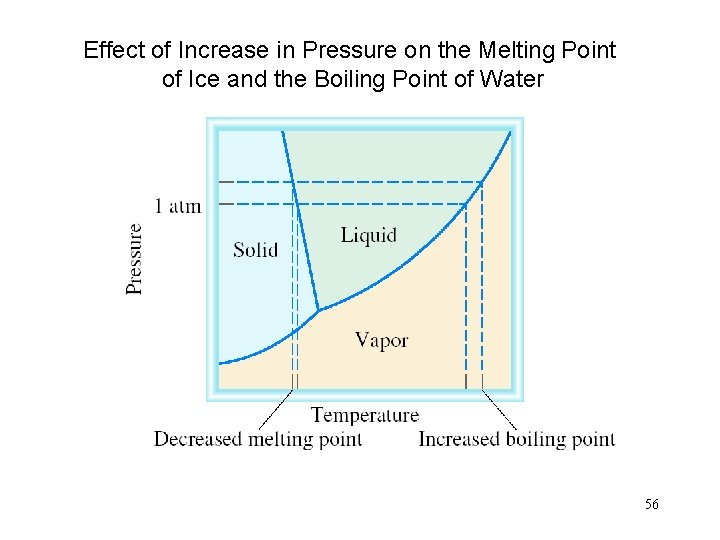Effect of Increase in Pressure on the Melting Point of Ice and the Boiling Point of Water 56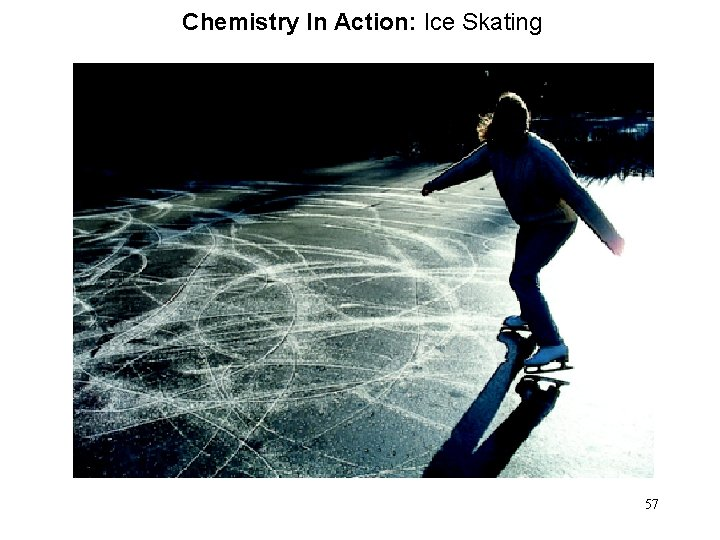Chemistry In Action: Ice Skating 57Chemistry In Action: Liquid Crystals 58